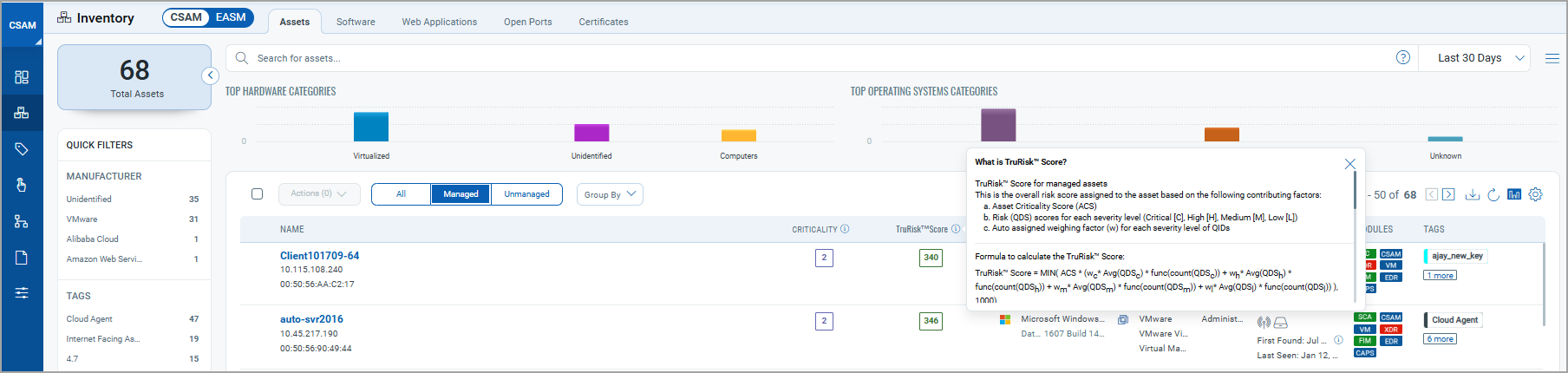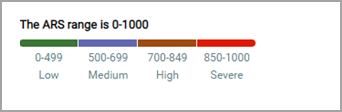# TruRisk Score for Assets

Learn more about the TruRisk Score for managed and externally exposed unmanaged assets. The TruRisk Score helps you prioritize the assets you should consider for VMDR activation.

TruRisk Score for Managed Assets

TruRisk Score for Externally Exposed Unmanaged Assets

## TruRisk Score for Managed Assets

The TrueRisk Score for managed assets is calculated based on the Asset Criticality Score (ACS) and Qualys Detection Score (QDS) assigned to vulnerabilities.

Complete the following steps to see the TruRisk formula and other required details:

1.  Go to the EASM tab and click Managed assets.
2.  Click the info icon from the TruRisk SCORE column. Detailed information about how the TruRisk Score is calculated for managed assets is shown.## TruRisk Score for Externally Exposed Unmanaged Assets

The TrueRisk Score for externally exposed unmanaged assets is calculated based on the Asset Criticality Score (ACS) and Qualys Vulnerability Score (QVS). The QVS is calculated based on vulnerabilities that are reported by Shodan.

Note: The TruRisk Score for externally exposed unmanaged assets is weighted 20% more than the managed assets.

Complete the following steps to see the TruRisk formula and other required details:

1.  Go to the EASM tab and click Unmanaged assets.
2.  Click the info icon from the TruRisk SCORE column. Detailed information about how the TruRisk Score is calculated for externally exposed unmanaged assets is shown.## TruRisk Score Formula for Managed and Externally Exposed Unmanaged Assets

What is TruRisk Score?

TruRisk Score for managed assets

This is the overall risk score assigned to the asset based on the following contributing factors:

a.  Asset Criticality Score (ACS)
b.  Risk (QDS) scores for each severity level (Critical [C], High [H], Medium [M], Low [L])
c.  Auto assigned weighing factor (w) for each severity level of QIDs

Formula to calculate the TruRisk Score:

TruRisk Score = MIN( ACS * (wc* Avg(QDSc) * np.power(Count(QDSc), 1/100) + wh* Avg(QDSh) * np.power(Count(QDSh), 1/100) + wm* Avg(QDSm) * np.power(Count(QDSm), 1/100) + wl* Avg(QDSl) * np.power(Count(QDSl), 1/100) ), 1000)

Where, w - weighing factor for each severity level of QIDs

Avg(QDS) - Average of Qualys detection score for each severity level of QIDs

If an asset doesn't have a critical vulnerability, the next available QDS will be used to calculate the TruRisk Score.

TruRisk Score for externally exposed unmanaged assets

This is the overall risk score assigned to External Attack Surface discovered unmanaged assets based on the following contributing factors:

a. Asset Criticality Score (ACS)
b. QVS scores for each severity level (Critical [C], High [H], Medium [M], Low [L])
c. Auto assigned weighing factor (w) for each severity level of QVS

Formula to calculate the TruRisk Score:

TruRisk Score = MIN( (Asset exposure) * ACS * (wc* Avg(QVSc) * np.power(Count(QVSc), 1/100) + wh* Avg(QVSh) * np.power(Count(QVSh), 1/100) + wm* Avg(QVSm) * np.power(Count(QVSm), 1/100) + wl* Avg(QVSl) * np.power(Count(QVSl), 1/100) ), 1000)

Where, w - weighing factor for each severity level of QVS

Avg(QVS) - Average of Qualys vulnerability score for each severity level of QVS